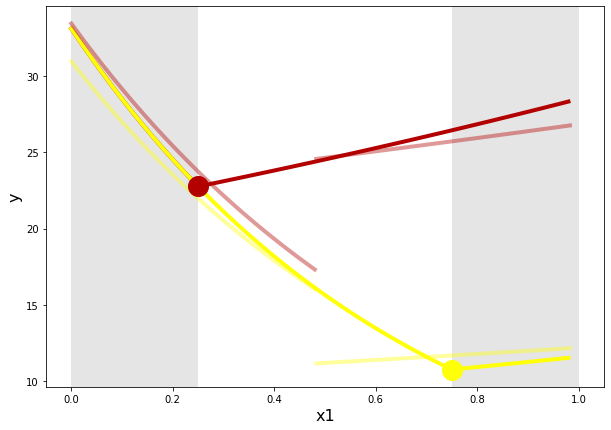# Fitting different models to parametric switchpoint data¶

In :
import numpy as np
import matplotlib
import matplotlib.pyplot as plt
import pandas as pd
%matplotlib inline

In :
# model definitions

def switch(params, data):
intercept = params
slope = params
switchpoint = intercept + (slope * data['z1'])
switchpoint = np.clip(switchpoint, 0, 1)
return switchpoint

def parametric_switch_model(params, data):
# params = [preIntercept, preSlope, slopeDiff, switchIntercept, switchSlope]

intercept = params
slope = params
slopeDiff = params
switchPoint = switch(params, data)
isPost = data['x1'] > switchPoint

mu = intercept + (slope * data['x1']) + (isPost * (slopeDiff * (data['x1']-switchPoint)))

return np.exp(mu)

def linear_model(params, data):
# params = [preIntercept, preSlope]

intercept = params
slope = params

mu = intercept + (slope * data['x1'])

return np.exp(mu)

def linear_withcov_model(params, data):
# params = [preIntercept, preSlope1, preSlope2]

intercept = params
slope1 = params
slope2 = params

mu = intercept + (slope1 * data['x1']) + (slope2 * data['z1'])

return np.exp(mu)

def fixed_switch_model(params, data):
# params = [preIntercept, preSlope, slopeDiff, switchPoint]

intercept = params
slope = params
slopeDiff = params
switchPoint = params
isPost = data['x1'] > switchPoint

mu = intercept + (slope * data['x1']) + (isPost * (slopeDiff * (data['x1']-switchPoint)))

return np.exp(mu)


The data was previously generated by the parametric switch model defined above. X1 and Z1 each varied uniformly over [0, 1]. A total of 1000 data points were generated.

In :
df = pd.read_csv('data.csv')


### Parameter estimates¶

All models were fit to the data. The estimates of their parameters were then extracted by hand and are now here.

In :
# these are the parameter values that were used to generate the data in the first place
trueparams = [3.5, -1.5, 1.8, 0.25, 0.5]

# estimates
linearfits = [3.277, -0.549]
linearwithcovfits = [3.486, -0.552, -0.425]
fixedswitchfits = [3.483, -1.437, 1.753, 0.483]
#fixedswitchthencov
fixed_switch_then_cov_pre = [3.51, -1.372, -0.077]
fixed_switch_then_cov_post= [3.119, 0.171, -0.79]
parametricswitchfits = [3.491, -1.51, 1.842, 0.26, 0.466]


### Visualize the model fits¶

#### Ground Truth¶

I am only going to plot a couple levels of z1 because the plots are going to get somewhat cluttered. Given that z1 is uniformly distributed over [0, 1], I have selected z1=.3333 and z1=.666. Just keep in mind that the data reflect a parametric family of switchpoints of which we are only visualizing two.

In :
plt.figure(figsize=(10,7))
plt.xlabel('x1', fontsize=16)
plt.ylabel('y', fontsize=16)

# plot the simulated data
plt.scatter(df['x1'], df['y'], s=20, c=df['z1'], alpha=0.5, cmap='hot', zorder=1)

# true parametric switch model
xs = np.arange(0,1,.02)
for z in [.333,.666]:
zs = z * np.ones_like(xs)
ys = parametric_switch_model(trueparams, pd.DataFrame(data={'x1': xs, 'z1': zs}))
plt.plot(xs, ys, linewidth=4, c=matplotlib.cm.get_cmap('hot')(z), zorder=5)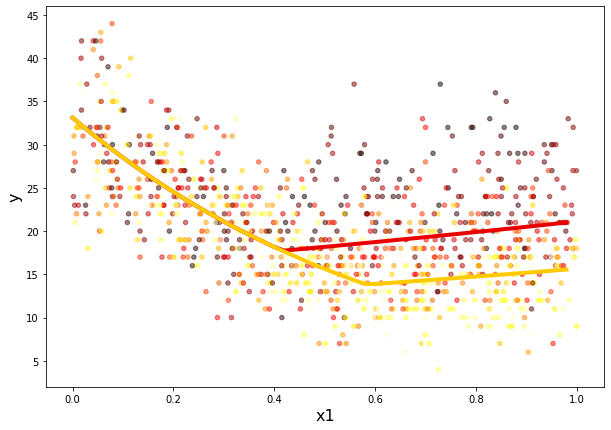### Linear model¶

This acts as a baseline. Nothing super interesting here.

In :
plt.figure(figsize=(10,7))
plt.xlabel('x1', fontsize=16)
plt.ylabel('y', fontsize=16)

# plot the simulated data
plt.scatter(df['x1'], df['y'], s=20, c=df['z1'], alpha=0.25, cmap='hot', zorder=1)

# true parametric switch model
xs = np.arange(0,1,.02)
for z in [.333,.666]:
zs = z * np.ones_like(xs)
ys = parametric_switch_model(trueparams, pd.DataFrame(data={'x1': xs, 'z1': zs}))
plt.plot(xs, ys, linewidth=4, c=matplotlib.cm.get_cmap('hot')(z), alpha = 0.5, zorder=5)

# linear model
ys = linear_model(linearfits, pd.DataFrame(data={'x1': xs}))
plt.plot(xs, ys, linewidth=4, c='k', zorder=5)

Out:
[<matplotlib.lines.Line2D at 0x7ff4a493b610>]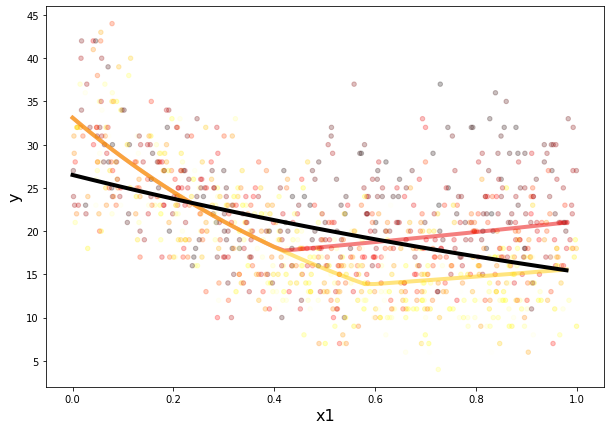#### Linear model with covariate(s) added¶

Here we add z1 to the model. Again, nothing too suprising.

In :
plt.figure(figsize=(10,7))
plt.xlabel('x1', fontsize=16)
plt.ylabel('y', fontsize=16)

# plot the simulated data
plt.scatter(df['x1'], df['y'], s=20, c=df['z1'], alpha=0.15, cmap='hot', zorder=1)

# true parametric switch model
xs = np.arange(0,1,.02)
for z in [.333,.666]:
zs = z * np.ones_like(xs)
ys = parametric_switch_model(trueparams, pd.DataFrame(data={'x1': xs, 'z1': zs}))
plt.plot(xs, ys, linewidth=4, c=matplotlib.cm.get_cmap('hot')(z), alpha = 0.333, zorder=5)

# linear model with covariates added
xs = np.arange(0,1,.02)
for z in [.333,.666]:
zs = z * np.ones_like(xs)
ys = linear_withcov_model(linearwithcovfits, pd.DataFrame(data={'x1': xs, 'z1': zs}))
plt.plot(xs, ys, linewidth=4, c=matplotlib.cm.get_cmap('hot')(z), zorder=5)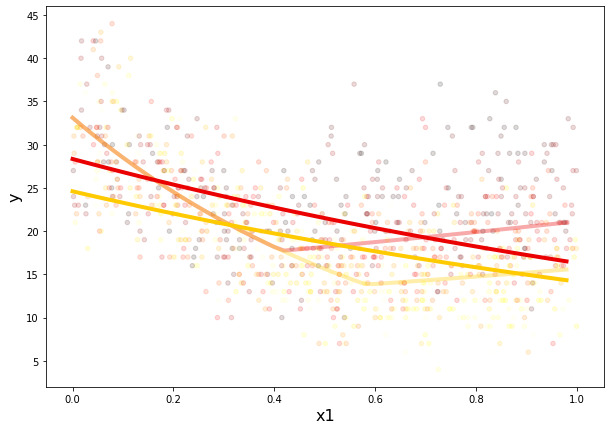#### Fixed switchpoint¶

Here we visualize the model in permits an estimate of a switchpoint, but assumes there is a single, global point at which teh change occurs (i.e., the point is not related to any covariates). Unsurprisinly, the fixed switchpoint model must split the difference between the true, low-z1 switchpoint and the true, high-z1 switchpoints.

In :
plt.figure(figsize=(10,7))
plt.xlabel('x1', fontsize=16)
plt.ylabel('y', fontsize=16)

# plot the simulated data
plt.scatter(df['x1'], df['y'], s=20, c=df['z1'], alpha=0.15, cmap='hot', zorder=1)

# true parametric switch model
xs = np.arange(0,1,.02)
for z in [.333,.666]:
zs = z * np.ones_like(xs)
ys = parametric_switch_model(trueparams, pd.DataFrame(data={'x1': xs, 'z1': zs}))
plt.plot(xs, ys, linewidth=4, c=matplotlib.cm.get_cmap('hot')(z), alpha = 0.333, zorder=5)

# fixed switchpoint
ys = fixed_switch_model(fixedswitchfits, pd.DataFrame(data={'x1': xs}))
plt.plot(xs, ys, linewidth=4, c='k', zorder=5)

print('Estimated switch point is ' + str(fixedswitchfits))

Estimated switch point is 0.483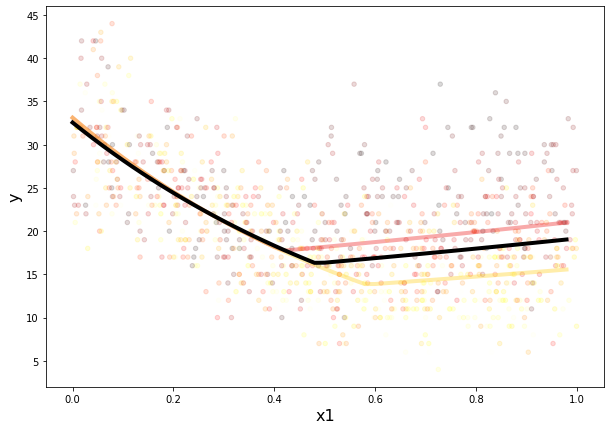#### Covariates explored after estimating a fixed switchpoint¶

Here, we assume the fixed switchpoint we just estimated. We then ask

1. whether our covariates (z1) account for any variance in the pre-switch regime
2. whether our covariates (z1) account for any variance in the post-switch regime
In :
plt.figure(figsize=(10,7))
plt.xlabel('x1', fontsize=16)
plt.ylabel('y', fontsize=16)

# plot the simulated data
plt.scatter(df['x1'], df['y'], s=20, c=df['z1'], alpha=0.15, cmap='hot', zorder=1)

# true parametric switch model
xs = np.arange(0,1,.02)
for z in [.333,.666]:
zs = z * np.ones_like(xs)
ys = parametric_switch_model(trueparams, pd.DataFrame(data={'x1': xs, 'z1': zs}))
plt.plot(xs, ys, linewidth=4, c=matplotlib.cm.get_cmap('hot')(z), alpha = 0.333, zorder=5)

# fixed switchpoint, then covariates added
fixed_switch = fixedswitchfits
# pre switch
xs = np.arange(0, fixed_switch, .02)
for z in [.333,.666]:
zs = z * np.ones_like(xs)
ys = linear_withcov_model(fixed_switch_then_cov_pre, pd.DataFrame(data={'x1': xs, 'z1': zs}))
plt.plot(xs, ys, linewidth=4, c=matplotlib.cm.get_cmap('hot')(z), zorder=5)
# post switch
xs = np.arange(fixed_switch, 1 ,.02)
for z in [.333,.666]:
zs = z * np.ones_like(xs)
ys = linear_withcov_model(fixed_switch_then_cov_post, pd.DataFrame(data={'x1': xs, 'z1': zs}))
plt.plot(xs, ys, linewidth=4, c=matplotlib.cm.get_cmap('hot')(z), zorder=5)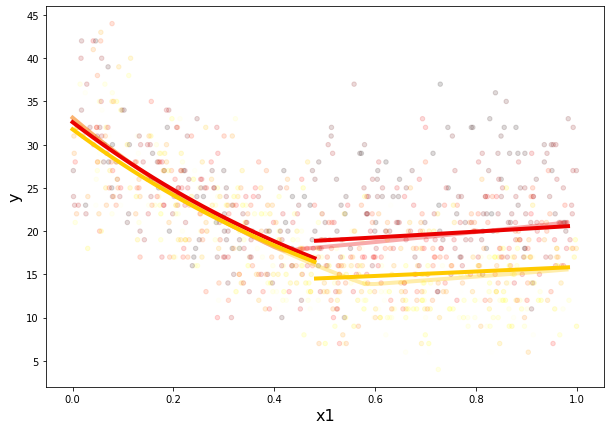These fits don't look terrible. This is, in part, because the misspecified fixed switchpoint model is misspecified in a way that means it will essentially be correct on average, but get the details wrong. Depending on the model parameters, these details may not matter much or they may be catstrophic. Let's zoom in to see exactly where the models diverge.

In :
f = plt.figure(figsize=(10,7))
f.gca().set_xlim([0.35, 0.65])
f.gca().set_ylim([10, 25])
plt.xlabel('x1', fontsize=16)
plt.ylabel('y', fontsize=16)

# true parametric switch model
xs = np.arange(0,1,.02)
parametric_switches = []
for z in [.333,.666]:
zs = z * np.ones_like(xs)
ys = parametric_switch_model(trueparams, pd.DataFrame(data={'x1': xs, 'z1': zs}))
parametric_switches += [trueparams + (trueparams* z)]
plt.plot(xs, ys, linewidth=4, c=matplotlib.cm.get_cmap('hot')(z), zorder=5)

trueswitch = switch(trueparams, pd.DataFrame(data={'x1': , 'z1': [z]}))
plt.plot(trueswitch, parametric_switch_model(trueparams, pd.DataFrame(data={'x1': trueswitch, 'z1': z})), \
c=matplotlib.cm.get_cmap('hot')(z), marker='o', ms=20, zorder=7)

# fixed switchpoint, then covariates added
fixed_switch = fixedswitchfits
# pre switch
xs = np.arange(0, fixed_switch, .02)
for z in [.333,.666]:
zs = z * np.ones_like(xs)
ys = linear_withcov_model(fixed_switch_then_cov_pre, pd.DataFrame(data={'x1': xs, 'z1': zs}))
plt.plot(xs, ys, linewidth=4, c=matplotlib.cm.get_cmap('hot')(z), alpha = 0.333, zorder=5)
# post switch
xs = np.arange(fixed_switch, 1 ,.02)
for z in [.333,.666]:
zs = z * np.ones_like(xs)
ys = linear_withcov_model(fixed_switch_then_cov_post, pd.DataFrame(data={'x1': xs, 'z1': zs}))
plt.plot(xs, ys, linewidth=4, c=matplotlib.cm.get_cmap('hot')(z), alpha = 0.333, zorder=5)

plt.axvspan(0, np.min(parametric_switches), facecolor='k', alpha=0.1)
plt.axvspan(np.max(parametric_switches), 1, facecolor='k', alpha=0.1)

Out:
<matplotlib.patches.Polygon at 0x7ff49ef87e90>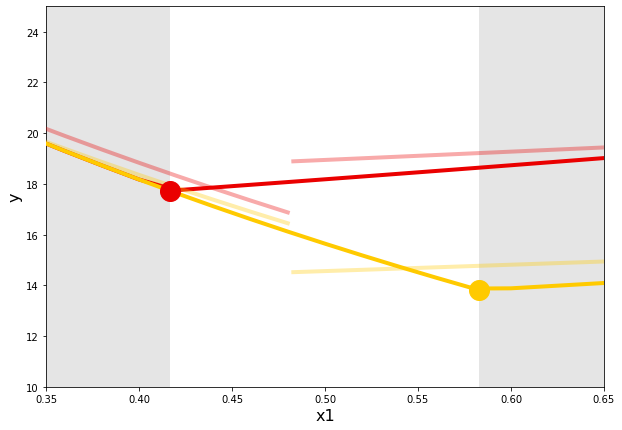In this plot we can see the divergence a bit more clearly (I hope). I have highlighted the parametric switchpoints for the two covariate values we are investigating here. I have done this both by placing data points at the exact location of the switchs and by 'greying out' the regions before and after the two relevant switchpoints.

As we saw earlier, the fixed switchpoint estimate is roughly in the middle of the true range, and this has two kinds of consequence. First, this switchpoint is an underestimate for some cases (red lines) and an overestimate for others (yellow). Within the interval the parameteric switchpoints cover (the white background), there an is obvious lack of agreement between the two models.

But I would also expect that the mis-estimated (really misspecified) switchpoint would systematically bias the pre- and post-switch parameter estimates as well. For example, considering values of the covariate for which the fixed switchpoint is an underestimate (yellow here), I would expect the post-switch intercept to be systematically overestimated and the post-switch slope to be underestimated. I would expect the opposite when considering values of the covariate for which the fixed switchpoint is an overestimate (red). The specific estimates here aren't entirely consistent with this expectation, but this is just one set of simulated data. I expect that they would be bourne out once we crank out a decently-sized set of simulated data (and associated parameter estimates).

#### Full range¶

Let's now take a look at a wider range of covariates (values of z1). In fact, let's look at the values that bracket the full interval over which z1 is distributed. Let's visualize z1=0 and z1=1.

In :
f = plt.figure(figsize=(10,7))
#f.gca().set_xlim([0.35, 0.65])
#f.gca().set_ylim([10, 25])
plt.xlabel('x1', fontsize=16)
plt.ylabel('y', fontsize=16)

# true parametric switch model
xs = np.arange(0,1,.02)
parametric_switches = []
zVals = [0, 1]
colorVals = [.25, .75]

for zIndex in [0, 1]:
zs = zVals[zIndex] * np.ones_like(xs)
ys = parametric_switch_model(trueparams, pd.DataFrame(data={'x1': xs, 'z1': zs}))
parametric_switches += [trueparams + (trueparams* zVals[zIndex])]
plt.plot(xs, ys, linewidth=4, c=matplotlib.cm.get_cmap('hot')(colorVals[zIndex]), zorder=5)

trueswitch = switch(trueparams, pd.DataFrame(data={'x1': , 'z1': [zVals[zIndex]]}))
plt.plot(trueswitch, parametric_switch_model(trueparams, pd.DataFrame(data={'x1': trueswitch, 'z1': zVals[zIndex]})), \
c=matplotlib.cm.get_cmap('hot')(colorVals[zIndex]), marker='o', ms=20, zorder=7)

# fixed switchpoint, then covariates added
fixed_switch = fixedswitchfits
# pre switch
xs = np.arange(0, fixed_switch, .02)
for zIndex in [0, 1]:
zs = zVals[zIndex] * np.ones_like(xs)
ys = linear_withcov_model(fixed_switch_then_cov_pre, pd.DataFrame(data={'x1': xs, 'z1': zs}))
plt.plot(xs, ys, linewidth=4, c=matplotlib.cm.get_cmap('hot')(colorVals[zIndex]), alpha = 0.4, zorder=5)
# post switch
xs = np.arange(fixed_switch, 1 ,.02)
for zIndex in [0, 1]:
zs = zIndex * np.ones_like(xs)
ys = linear_withcov_model(fixed_switch_then_cov_post, pd.DataFrame(data={'x1': xs, 'z1': zs}))
plt.plot(xs, ys, linewidth=4, c=matplotlib.cm.get_cmap('hot')(colorVals[zIndex]), alpha = 0.4, zorder=5)

plt.axvspan(0, np.min(parametric_switches), facecolor='k', alpha=0.1)
plt.axvspan(np.max(parametric_switches), 1, facecolor='k', alpha=0.1)

plt.savefig('widerZrange.png')